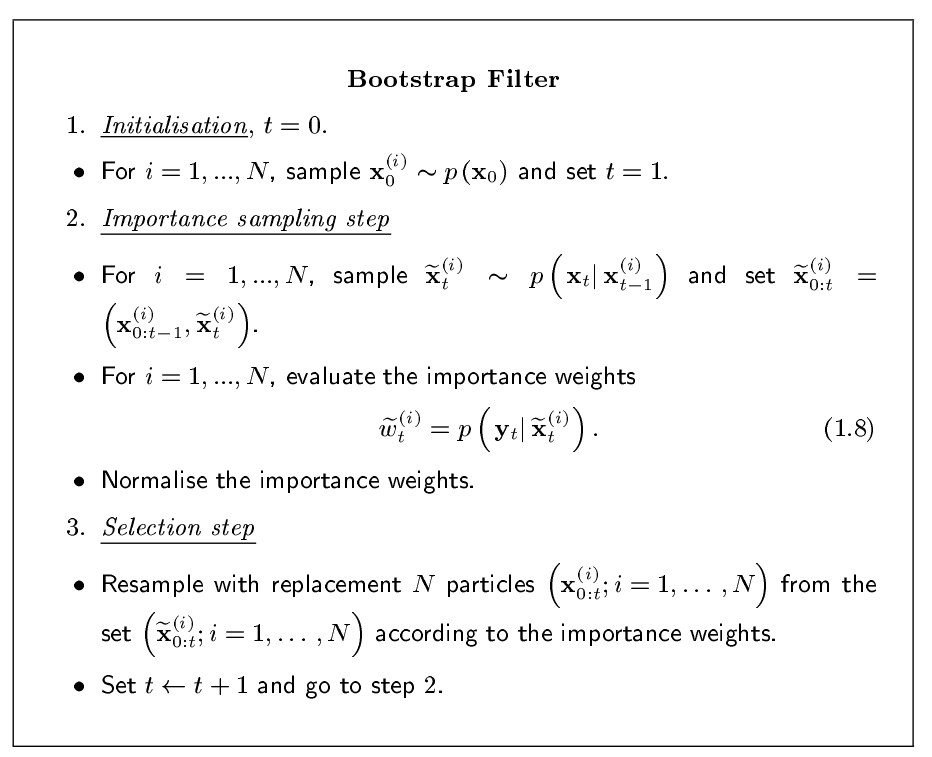# Sequential Monte Carlo Methods

##### Posted on Jun 10, 2017 (Update: Jan 31, 2019) 0 Comments

The first peep to SMC as an abecedarian, a more comprehensive note can be found here.

## Motivation

observations arrive sequentially in time and one is interested in performing inference on-line.

need to update the posterior distribution as data become available.

1. if the data are modelled by a linear Gaussian state-space model, it is possible to derive an exact analytical expression to compute the evolving sequence of posterior distributions. kalman filter.

2. if the data are modelled as a partially observed, finite state-space Markov chain, it is also possible to obtain an analytical solution, HMM filter

conditions which preclude analytic solution

1. non-Gaussianity
2. high dimensionality
3. nonlinearity

approximation schemes:

1. extended Kalman filter
2. Gaussian sum approximations
3. grid-based filters (based on deterministic numerical integration methods, difficult to implement and too expensive for high dimensions)

the first two methods fail to take into account all the salient statistical features of the processes under considerations, leading quite often to poor results.

Sequential Monte Carlo (SMC): a set of simulation-based methods which provides a convenient and attractive approach to computing the posterior distributions.

• flexible
• easy to implement
• parallelisable
• applicable in very general settings

## Problem statement

Considering Markovian, nonlinear, non-Gaussian state-space models, the unobserved signal (hidden states) $\{x_t;t\in N\}, x_t\in \cal X$, is modeled as a Markov process of initial distribution $p(x_0)$ and transition equation $p(x_t\mid x_{t-1})$. The observations $\{y_t;t\in N^*\}, y_t\in \cal Y$, are assumed to be conditionally independent given the process $\{x_t;t\in N\}$ and of marginal distribution $p(y_t\mid x_t)$.

aim: estimate recursively in time the posterior distribution $p(x_{0:t}\mid y_{1:t})$, its associated features, and the expectations

## Monte Carlo methods

### Perfect Monte Carlo sampling

Unfortunately, it is usually impossible to sample efficiently from the posterior distribution $p(x_{0:t}\mid y_{1:t})$ at time t.

MCMC are iterative algorithms unsuited to recursive estimation problems.

### Importance sampling

a general Monte Carlo integration method, but not adequate for recursive estimation.

### Sequential Importance Sampling

usually inefficient in high-dimensional spaces

as t increases, the distribution of the importance weights becomes more and more skewed. Partically, after a few time steps, only one particle has a non-zero importance weight.

### Notion

key idea: eliminate the particles having low importance weights and to multiply particles having high importance weights.attractive properties

1. very quick and easy to implement
2. to a large extent modular
3. implemented on a parallel computer
4. resampling step is a black box routine, only requires inputs

SMC_Resources